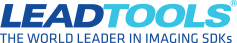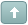Error

# HOW TO: Resize image and maintain aspect ratio (if needed)

#1 Posted : Wednesday, June 12, 2019 10:07:09 AM(UTC)
If you need to determine if an image needs to be resized to fit within a rectangle, then you can use the following method:

Code:
``````      public static void ResizeAspectRatio(RasterImage img, int canvasWidth, int canvasHeight)
{
// image is smaller than the canvas so no need to resize
if (img.Width < canvasWidth && img.Height < canvasHeight)
return;

// Figure out the ratio
double ratioX = (double)canvasWidth / (double)img.Width;
double ratioY = (double)canvasHeight / (double)img.Height;
// use whichever multiplier is smaller
double ratio = ratioX < ratioY ? ratioX : ratioY;

// now we can get the new height and width
int newHeight = Convert.ToInt32(img.Height * ratio);
int newWidth = Convert.ToInt32(img.Width * ratio);

//Resize the image
SizeCommand sizeCommand = new SizeCommand(newWidth, newHeight, RasterSizeFlags.Resample);
sizeCommand.Run(img);
}``````
Developer Support ManagerTry the latest version of LEADTOOLS for free for 60 days by downloading the evaluation: https://www.leadtools.com/downloadsWanna join the discussion? Login to your LEADTOOLS Support accountor Register a new forum account.